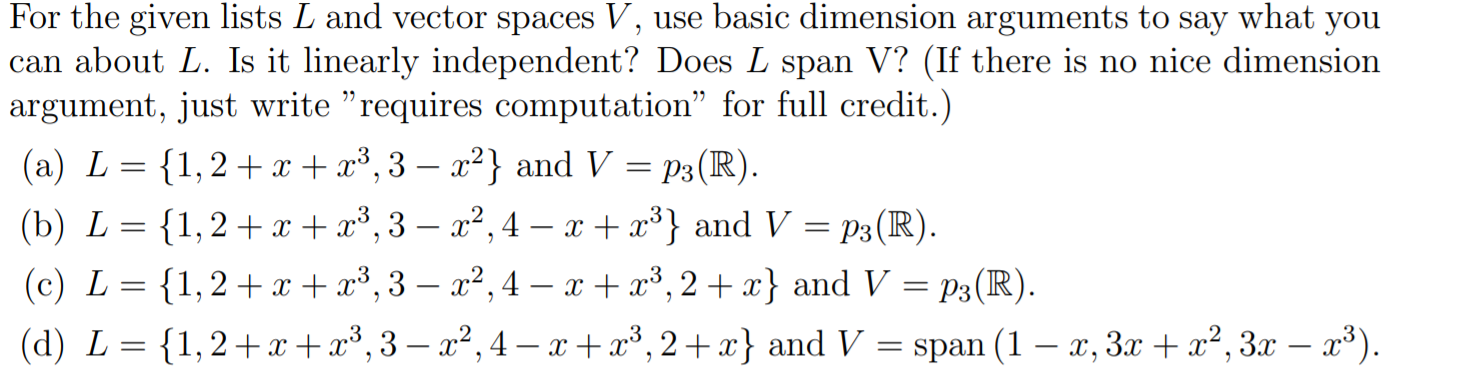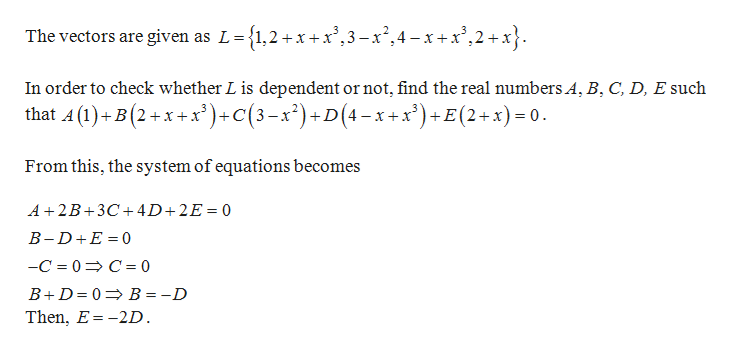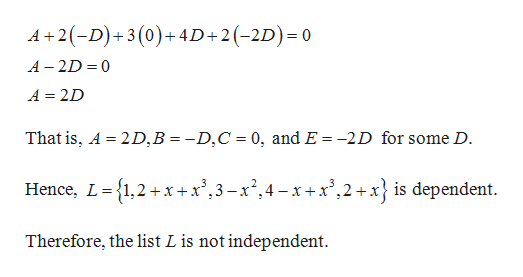# For the given lists L and vector spaces V, use basic dimension arguments to say what you| can about L. Is it linearly independent? Does L span V? (If there is no nice dimensionargument, just write "requires computation" for full credit.)(a) L {1,2x3,3 - a2} and V p3(R)1,2 x, - a2,4 - x 3} and V = p3(R).(b) L(c) L {1,2 x x3,3- 2,4 - x + x3, 2+x} and V = p3(R)1,2 x3,-x2,4 -x+x3,2+ x} and V = span (1 - x, 3x x2, 3x - 3)|(d) L

Question
60 viewshelp_outlineImage TranscriptioncloseFor the given lists L and vector spaces V, use basic dimension arguments to say what you | can about L. Is it linearly independent? Does L span V? (If there is no nice dimension argument, just write "requires computation" for full credit.) (a) L {1,2x3,3 - a2} and V p3(R) 1,2 x, - a2,4 - x 3} and V = p3(R). (b) L (c) L {1,2 x x3,3- 2,4 - x + x3, 2+x} and V = p3(R) 1,2 x3,-x2,4 -x+x3,2+ x} and V = span (1 - x, 3x x2, 3x - 3) |(d) L fullscreen
check_circle

Step 1

Given:help_outlineImage TranscriptioncloseL- {1,2+x+x,3-x,4-xx,2+x} The vectors are given _ In order to check whether L is dependent or not, find the real numbers A, B, C, D, E such that A (1)+B(2+x+x')+C(3-x2)+D(4 x+x')+E(2+x) 0 From this, the system of equations becomes A 2B 3C4D+2E 0 B-D E 0 -C 0 C 0 B+D 0 B = -D Then, E-2D fullscreen
Step 2

For some D,

...help_outlineImage TranscriptioncloseA+2D)+3 (0)+4D+2(-2D) 0 = A 2D 0 A 2D That is, A 2D,B = -D,C 0, and E = -2D for some D. Hence, L 1,2+x+x',3-x2,4-x+x,2+x} is dependent. = Therefore, the list L is not independent fullscreen

### Want to see the full answer?

See Solution

#### Want to see this answer and more?

Solutions are written by subject experts who are available 24/7. Questions are typically answered within 1 hour.*

See Solution
*Response times may vary by subject and question.
Tagged in

### Math